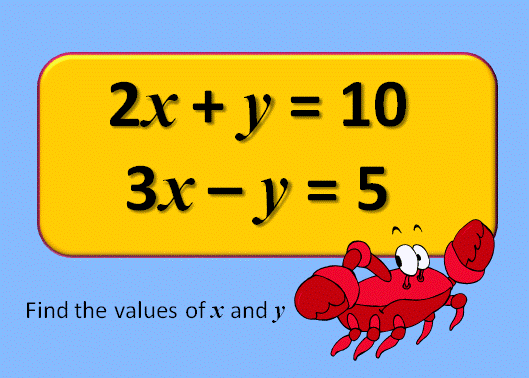and

This is question number 1

Simultaneous equations are two equations with two unknowns. They are called simultaneous because they must both be solved at the same time. Have a look at some of these problems which lead to simultaneous equations:

There are also a series of randomly-generated, self-marking simultaneous equations exercises you clould try:

There are solutions to this exercise but they are only available to those who have a Transum Subscription.For All: